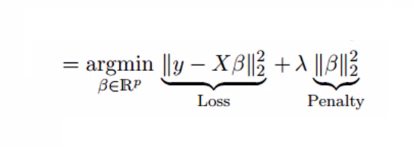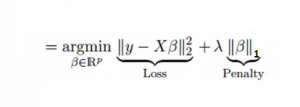# Ridge Regression and Lasso Regression

Ridge Regression is a technique used when the data suffers from multicollinearity (independent variables are highly correlated). In multicollinearity, even though the least squares estimates (OLS) are unbiased, their variances are large which deviates the observed value far from the true value. By adding a degree of bias to the regression estimates, ridge regression reduces the standard errors.

Above, we saw the equation for linear regression. Remember? It can be represented as:

y=a+ b*x

This equation also has an error term. The complete equation becomes:

y=a+b*x+e (error term), [error term is the value needed to correct for a prediction error between the observed and predicted value]

=> y=a+y= a+ b1x1+ b2x2+…+e, for multiple independent variables.

In a linear equation, prediction errors can be decomposed into two sub components. First is due to the biased and second is due to the variance . Prediction error can occur due to any one of these two or both components. Here, we’ll discuss about the error caused due to variance.

Ridge regression solves the multicollinearity problem through shrinkage parameter λ (lambda). Look at the equation below.In this equation, we have two components. First one is least square term and other one is lambda of the summation of β2 (beta- square) where β is the coefficient. This is added to least square term in order to shrink the parameter to have a very low variance.

#### Important Points:

• The assumptions of this regression is same as least squared regression except normality is not to be assumed
• Ridge regression shrinks the value of coefficients but doesn’t reaches zero, which suggests no feature selection feature
• This is a regularization method and uses l2 regularization.

## 6. Lasso RegressionSimilar to Ridge Regression, Lasso (Least Absolute Shrinkage and Selection Operator) also penalizes the absolute size of the regression coefficients. In addition, it is capable of reducing the variability and improving the accuracy of linear regression models. Look at the equation below: Lasso regression differs from ridge regression in a way that it uses absolute values in the penalty function, instead of squares. This leads to penalizing (or equivalently constraining the sum of the absolute values of the estimates) values which causes some of the parameter estimates to turn out exactly zero. Larger the penalty applied, further the estimates get shrunk towards absolute zero. This results to variable selection out of given n variables.

#### Important Points:

• The assumptions of lasso regression is same as least squared regression except normality is not to be assumed
• Lasso Regression shrinks coefficients to zero (exactly zero), which certainly helps in feature selection
• Lasso is a regularization method and uses l1 regularization
• If group of predictors are highly correlated, lasso picks only one of them and shrinks the others to zero